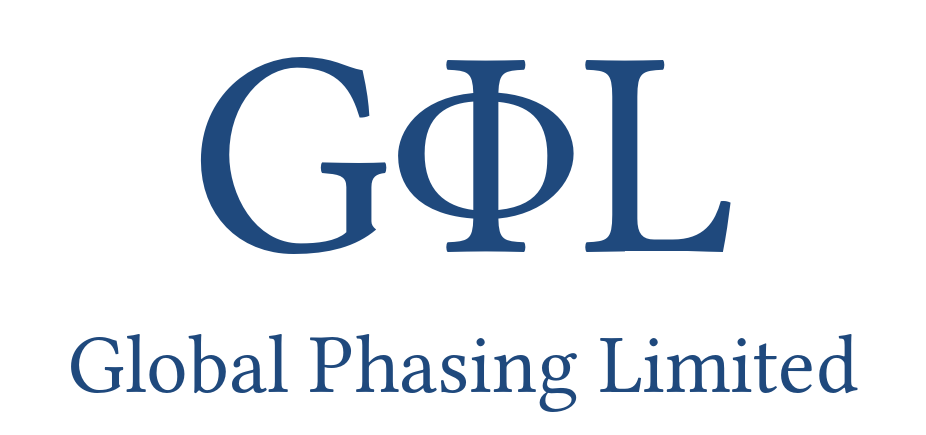## The STARANISO Server

### Anisotropy of the Diffraction LimitandBayesian Estimation of Structure Amplitudes### GLOSSARY of terminology related to anisotropy:

 Isotropy The property of being directionally independent, implying no variation of specified physical properties with direction. Anisotropy The property of being directionally dependent, implying variation of specified physical properties with direction. Diffraction limit The isotropic or anisotropic limit on average of statistically significant data (also known as the 'resolution limit'), depending on both the properties of the crystal and the data-collection strategy. Threshold Criterion used to make a decision on rejection of data. Spherical shell Region of reciprocal space lying between two defined reciprocal-space radii (d*).  The statistics of both isotropic and anisotropic properties are analyzed in spherical shells. Diffraction cut-off Cut-off applied to all diffraction data outside a single closed isotropic or anisotropic surface in reciprocal space defining the assumed boundary between, on average, statistically significant and insignificant data.  In the case of an isotropic surface only one parameter is needed to define it, i.e. the radius of the limiting sphere.  In the general case the surface is anisotropic and one parameter (or even a small number of parameters) is not sufficient. Possible data All reflections inside the greatest value of d* for diffraction cut-offs in all directions, regardless of whether or not an intensity was measured (systematic absences are excluded everywhere). Measured data All possible reflections with an experimental intensity and standard uncertainty. Unmeasured data All possible reflections without an experimental intensity and standard uncertainty. Observed data All measured reflections inside the diffraction cut-off surface and included in the statistical tables and the reflection output file. Unobserved data All measured reflections outside the diffraction cut-off surface and excluded from the statistical tables and the reflection output file. Observable data All unmeasured reflections inside the diffraction cut-off surface (i.e. expected to be observed). Unobservable data All unmeasured reflections outside the diffraction cut-off surface (i.e. expected to be unobserved). Fitted ellipsoid Ellipsoid fitted by a multi-dimensional search and weighted least squares algorithm to the reflection data immediately neighboring the diffraction cut-off surface.  Used to reduce the number of parameters required to define the anisotropic diffraction cut-off surface and thereby estimate the anisotropic diffraction limits and predict observable data. Debye-Waller factor Attenuation factor of each measured intensity and its standard uncertainty due to thermal motion and/or static disorder: exp(-4 π2 shT U sh) , where sh is the reciprocal-lattice (column) vector, shT is the same as a row vector and U is the overall anisotropy tensor (= B / 8 π2). Intensity prior Expected intensity, estimated from a spherical intensity distribution obtained from experimental data or from a model, combined with the symmetry enhancement factor and the isotropic or anisotropic Debye-Waller factor. Anisotropy correction factor The inverse of the square root of the anisotropic Debye-Waller factor applied as a multiplicative correction of the amplitudes after Bayesian estimation from the intensities:  exp(2 π2 shT ΔU sh) where ΔU is the overall anisotropy U tensor after subtraction of Ueq from its diagonal elements, where Ueq is the isotropic equivalent U (mean of the eigenvalues), so that the trace of ΔU is zero. Completeness Number of data as a fraction of the number of possible data in any defined volume of reciprocal space (e.g. within the intersection of a spherical shell with the fitted ellipsoid). Anisotropy ratio Difference between the largest and smallest eigenvalues of the overall anisotropy U tensor divided by Ueq : (Emax - Emin) / Ueq.  This metric lies between zero (isotropic) and 3 (maximally anisotropic). Fractional anisotropy The RMS deviation of the eigenvalues from the mean, scaled to lie between zero and one: √(1.5 Σi (Ei - Ueq)2 / Σi Ei2).  Unlike the anisotropy ratio this metric takes into account all 3 eigenvalues. Anisotropic S/N ratio The maximum value over all observed reflections of the absolute deviation of the squared anisotropy correction factor from 1, multiplied by the local mean intensity / standard uncertainty ratio: Maxh (| exp(4 π2 shT ΔU sh) - 1 | ) .  Unlike the anisotropy ratio and the fraction anisotropy this takes into account both the fact that large anisotropies represent larger differences in intensity at high d* and that there will be a greater contribution from reflections with a high value of the local mean intensity / standard uncertainty ratio.  This metric is zero in the isotropic case, with no limit in the anisotropic case. Weighted CC½ The inverse-variance-weighted half-dataset Pearson product-moment correlation coefficient, where the variances are those of the overall inverse-variance-weighted mean intensities.  If the half-dataset inverse-variance-weighted mean intensities are available (e.g. from MRFANA), this is computed using the standard formula for the weighted correlation coefficient.  If the half-dataset weighted mean intensities are not available it is computed using a modification (i.e. using inverse-variance weighting as above) of the 'σ-τ' method of Assmann et al. Fisher transformation of the weighted CC½ This uses the standard formula. Kullback-Leibler divergence (a.k.a. 'relative entropy') This uses the standard formula with logarithms base 10 (so its unit is the 'hartley' or 'ban').